# MCAT Physical : Poiseuille Flow and Turbulence

## Example Questions

### Example Question #1 : Poiseuille Flow And Turbulence

Diffusion can be defined as the net transfer of molecules down a gradient of differing concentrations. This is a passive and spontaneous process and relies on the random movement of molecules and Brownian motion. Diffusion is an important biological process, especially in the respiratory system where oxygen diffuses from alveoli, the basic unit of lung mechanics, to red blood cells in the capillaries.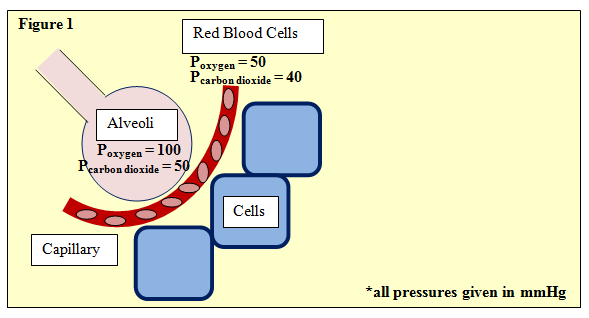Figure 1 depicts this process, showing an alveoli separated from neighboring cells by a capillary with red blood cells. The partial pressures of oxygen and carbon dioxide are given. One such equation used in determining gas exchange is Fick's law, given by:

ΔV = (Area/Thickness) · Dgas · (P1 – P2)

Where ΔV is flow rate and area and thickness refer to the permeable membrane through which the gas passes, in this case, the wall of the avlveoli. P1 and P2 refer to the partial pressures upstream and downstream, respectively. Further, Dgas­, the diffusion constant of the gas, is defined as:

Dgas = Solubility / (Molecular Weight)^(1/2)

During strenuous exercise, blood flow greatly increases. Compared to red blood cells traveling along the sides of the capillary, how will oxygen diffusion be affected for those red blood cells traveling towards the center of the capillary?

Lower diffusion towards the periphery

Higher diffusion towards the center

No change

Lower diffusion towards the center

Lower diffusion towards the center

Explanation:

This is another question testing fluid dynamics. In any fluid, less resistance will be encountered towards the center of the vessel; therefore, red blood cells travelling in the center of the capillary will be travelling faster and have less time to "catch" oxygen as it diffuses towards the blood stream. Interestingly, this same feature also helps to explain why damaged or cut vessels clot so quickly—clotting factors, such as platelets, towards the periphery move more slowly, thus bunching up near the wound site.

### Example Question #2 : Poiseuille Flow And Turbulence

Use the following information to answer questions 1-6:

The circulatory system of humans is a closed system consisting of a pump that moves blood throughout the body through arteries, capillaries, and veins. The capillaries are small and thin, allowing blood to easily perfuse the organ systems. Being a closed system, we can model the human circulatory system like an electrical circuit, making modifications for the use of a fluid rather than electrons. The heart acts as the primary force for movement of the fluid, the fluid moves through arteries and veins, and resistance to blood flow occurs depending on perfusion rates.

To model the behavior of fluids in the circulatory system, we can modify Ohm’s law of V = IR to ∆P = FR where ∆P is the change in pressure (mmHg), F is the rate of flow (ml/min), and R is resistance to flow (mm Hg/ml/min). Resistance to fluid flow in a tube is described by Poiseuille’s law: R = 8hl/πr4 where l is the length of the tube, h is the viscosity of the fluid, and r is the radius of the tube. Viscosity of blood is higher than water due to the presence of blood cells such as erythrocytes, leukocytes, and thrombocytes.

The above equations hold true for smooth, laminar flow. Deviations occur, however, when turbulent flow is present. Turbulent flow can be described as nonlinear or tumultuous, with whirling, glugging or otherwise unpredictable flow rates. Turbulence can occur when the anatomy of the tube deviates, for example during sharp bends or compressions. We can also get turbulent flow when the velocity exceeds critical velocity vc, defined below.

vc = NRh/ρD

NR is Reynold’s constant, h is the viscosity of the fluid, ρ is the density of the fluid, and D is the diameter of the tube. The density of blood is measured to be 1060 kg/m3.

Another key feature of the circulatory system is that it is set up such that the organ systems act in parallel rather than in series. This allows the body to modify how much blood is flowing to each organ system, which would not be possible under a serial construction. This setup is represented in Figure 1.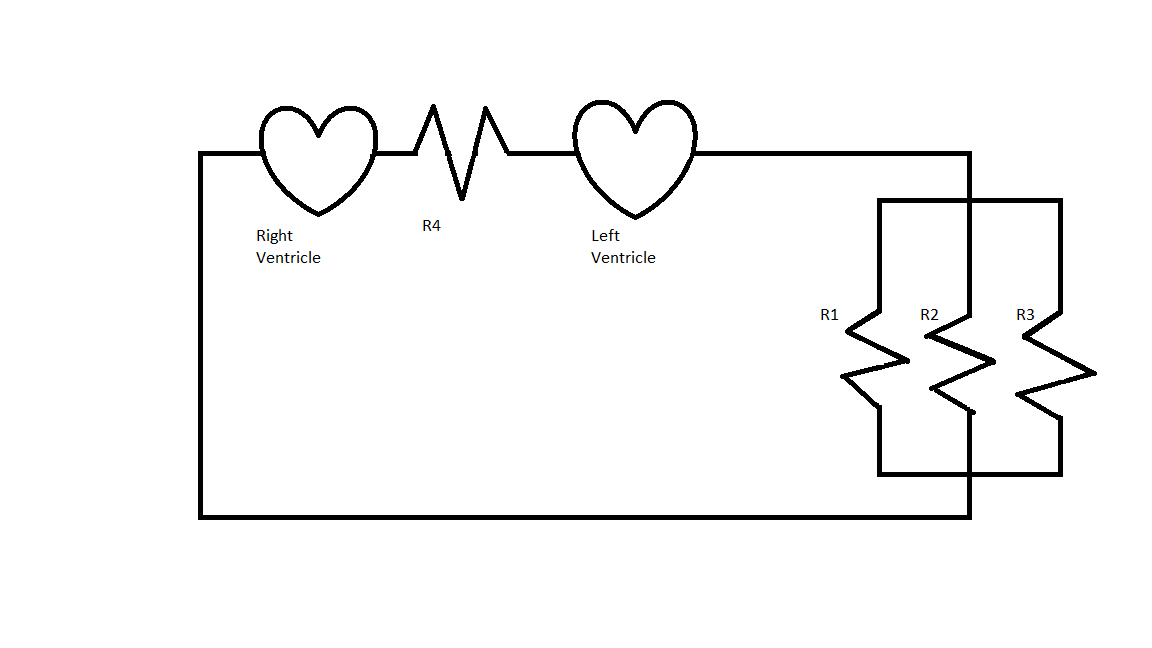Which of the following disorders would most likely cause an increase in turbulent flow?

I. Increased cardiac output

II. Anemia

III. Lung cancer

II only

I and II

II and III

I only

I and II

Explanation:

We can see from the equation that critical velocity depends on Reynolds number, viscosity, diameter of the tube, and density of the fluid. If we assume the heart is pumping normally and that the velocity of blood is constant, a decrease in the critical velocity would increase the chance that the normal velocity would exceed this critical velocity. This would result in turbulent flow.

Looking at the choices, anemia would cause a decrease in blood viscosity, resulting in a lower critical velocity, increasing the chance for turbulent flow. Increased cardiac output would increase the average velocity of the blood such that it may overcome the critical velocity, resulting in more turbulence. Lung cancer should not have a measurable effect on blood turbulence.

### Example Question #3 : Poiseuille Flow And Turbulence

Use the following information to answer questions 1-6:

The circulatory system of humans is a closed system consisting of a pump that moves blood throughout the body through arteries, capillaries, and veins. The capillaries are small and thin, allowing blood to easily perfuse the organ systems. Being a closed system, we can model the human circulatory system like an electrical circuit, making modifications for the use of a fluid rather than electrons. The heart acts as the primary force for movement of the fluid, the fluid moves through arteries and veins, and resistance to blood flow occurs depending on perfusion rates.

To model the behavior of fluids in the circulatory system, we can modify Ohm’s law of V = IR to ∆P = FR where ∆P is the change in pressure (mmHg), F is the rate of flow (ml/min), and R is resistance to flow (mm Hg/ml/min). Resistance to fluid flow in a tube is described by Poiseuille’s law: R = 8hl/πr4 where l is the length of the tube, h is the viscosity of the fluid, and r is the radius of the tube. Viscosity of blood is higher than water due to the presence of blood cells such as erythrocytes, leukocytes, and thrombocytes.

The above equations hold true for smooth, laminar flow. Deviations occur, however, when turbulent flow is present. Turbulent flow can be described as nonlinear or tumultuous, with whirling, glugging or otherwise unpredictable flow rates. Turbulence can occur when the anatomy of the tube deviates, for example during sharp bends or compressions. We can also get turbulent flow when the velocity exceeds critical velocity vc, defined below.

vc = NRh/ρD

NR is Reynold’s constant, h is the viscosity of the fluid, ρ is the density of the fluid, and D is the diameter of the tube. The density of blood is measured to be 1060 kg/m3.

Another key feature of the circulatory system is that it is set up such that the organ systems act in parallel rather than in series. This allows the body to modify how much blood is flowing to each organ system, which would not be possible under a serial construction. This setup is represented in Figure 1.Which of the following accurately demonstrates a relationship between critical velocity and resistance?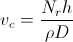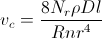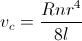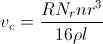Explanation:

Viscosity (h) is common to both equations, so to find a relationship between resistance and critical velocity, we can use the resistance equation and solve for viscosity.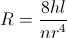andRearranging the equation for resistance allows us to substitute for h in the critical velocity equation.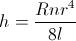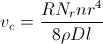This equation can be further reduced. Diameter is twice the radius, so D = 2r.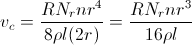### Example Question #4 : Poiseuille Flow And Turbulence

Diffusion can be defined as the net transfer of molecules down a gradient created by differing concentrations of the molecule in different locations. This is a passive, spontaneous process and relies on the random movement of molecules and Brownian motion. Diffusion is an important biological process, especially in the respiratory system where oxygen diffuses from alveoli, the basic units of lung mechanics, to red blood cells in the capillaries.Figure 1 depicts this process, showing an alveolus separated from neighboring cells by a capillary with red blood cells. The partial pressures of oxygen and carbon dioxide are given. One equation used in determining gas exchange is Fick's law, given by: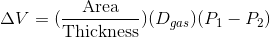In this equation,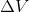is the flow rate. Area and thickness refer to the permeable membrane through which the gas passesin this case, the wall of the alveolus.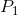and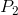refer to the partial pressures upstream and downstream, respectively.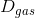, the diffusion constant of the gas, is defined as:During inspiration, the diaphragm contracts and allows oxygen to rush into the lungs. How would a change in average airway diameter affect the speed at which oxygen moves?

Increase in airway diameter would decrease oxygen speed

Oxygen speed during inspiration remains constant, regardless of airway diameter

A decrease in airway diameter would decrease oxygen speed

A decrease in airway diameter would increase oxygen speed

Airway diameter will not impact oxygen speed, but will impact inspiration volume

A decrease in airway diameter would decrease oxygen speed

Explanation:

Note, two of the choices say essentially say the same relationship. Therefore, one can immediately infer that they are wrong. The question is based off of knowledge of fluid dynamics. Typically, a decrease in airway diameter will provide more resistance and thus lower the speed at which a fluid (or gas) will move. Think about blowing air through a series of straws of narrowing diameter; the narrower the straw, the harder it is to blow air; therefore, a decrease in airway diameter would cause an decrease in oxygen speed.

### Example Question #5 : Poiseuille Flow And Turbulence

Use the following information to answer questions 1-6:

The circulatory system of humans is a closed system consisting of a pump that moves blood throughout the body through arteries, capillaries, and veins. The capillaries are small and thin, allowing blood to easily perfuse the organ systems. Being a closed system, we can model the human circulatory system like an electrical circuit, making modifications for the use of a fluid rather than electrons. The heart acts as the primary force for movement of the fluid, the fluid moves through arteries and veins, and resistance to blood flow occurs depending on perfusion rates.

To model the behavior of fluids in the circulatory system, we can modify Ohm’s law of V = IR to ∆P = FR where ∆P is the change in pressure (mmHg), F is the rate of flow (ml/min), and R is resistance to flow (mm Hg/ml/min). Resistance to fluid flow in a tube is described by Poiseuille’s law: R = 8hl/πr4 where l is the length of the tube, h is the viscosity of the fluid, and r is the radius of the tube. Viscosity of blood is higher than water due to the presence of blood cells such as erythrocytes, leukocytes, and thrombocytes.

The above equations hold true for smooth, laminar flow. Deviations occur, however, when turbulent flow is present. Turbulent flow can be described as nonlinear or tumultuous, with whirling, glugging or otherwise unpredictable flow rates. Turbulence can occur when the anatomy of the tube deviates, for example during sharp bends or compressions. We can also get turbulent flow when the velocity exceeds critical velocity vc, defined below.

vc = NRh/ρD

NR is Reynold’s constant, h is the viscosity of the fluid, ρ is the density of the fluid, and D is the diameter of the tube. The density of blood is measured to be 1060 kg/m3.

Another key feature of the circulatory system is that it is set up such that the organ systems act in parallel rather than in series. This allows the body to modify how much blood is flowing to each organ system, which would not be possible under a serial construction. This setup is represented in Figure 1.One of the effects of norepinephrine is vasoconstriction of peripheral blood vessels. If the medication reduces the diameter of a peripheral vessel in the hands by ½, what effect does this have on the flow of blood to hands?

Flow will increase by a factor of 16

Flow will decrease by a factor of 16

Flow will decrease by a factor of 8

Flow will increase by a factor of 8

Flow will decrease by a factor of 16

Explanation:

Resistance is measured by the equation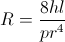.

If the diameter is reduced by half, the radius is reduced by half. This will increase resistance by a factor of 24, or 16. We know that the circulatory system is set up as a parallel system so the pressure in any branch must be constant, allowing only flow to change. Using the equation ∆P = FR, if resistance increases by a factor of 16 and the flow stays the same, then flow decreases by a factor of 16 since pressure is constant.

∆P = F2R2 – F1R1 = 0

16R1 = R2

F2R2 = F1R1

F216R1 = F1R1

F2= (1/16)F1

### Example Question #6 : Poiseuille Flow And Turbulence

Which change will produce the greatest increase to flow rate through a tube?

Doubling the pressure

Halving the length of the tube while tripling the pressure

Tripling the length of the tube while halving the pressure

Explanation:

The equation for flow rate through a section of tube is given by Poiseulle's law. Though you will not need to memorize this equation, you must be familiar with the relationships it describes on a conceptual basis.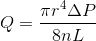In this equation,is the radius,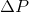is the change in pressure,is the liquid viscosity, andis the length of the tube.

Of the given answer option, doubling the radius would multiply the flow rate by, resulting in the greatest increase.

### Example Question #7 : Poiseuille Flow And Turbulence

A variation of Poiseuille's law, a relationship that helps explain fluid resistance in a pipe, is given by: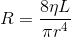In the formula,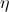is viscosity,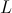is the length of the pipe, and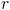is the diameter of the pipe.

Which one of the following effects would decrease resistance?

Narrowing the pipe

Decreasing flow

Increasing fluid temperature

Extending the pipe

Increasing fluid pressure

Increasing fluid temperature

Explanation:

This question can be answered quickly from logic, with little math involved. Temperature itself is not a direct variable in the given equation; however, it does relate to viscosity,. The warmer a fluid is, the lower the viscosity will become, and a fluid that is less viscous will flow more easily, creating less resistance.

The other answer choices either give changes that are unrelated to flow resistance, or increase flow resistance. Narrowing the pipe and increasing the length would increase resistance, according to the given equation. Flow and pressure can be affected by resistance, but cannot change the given resistance in a system.

### Example Question #8 : Poiseuille Flow And Turbulence

Diffusion can be defined as the net transfer of molecules down a gradient created by differing concentrations of the molecule in different locations. This is a passive, spontaneous process and relies on the random movement of molecules and Brownian motion. Diffusion is an important biological process, especially in the respiratory system where oxygen diffuses from alveoli, the basic units of lung mechanics, to red blood cells in the capillaries.Figure 1 depicts this process, showing an alveolus separated from neighboring cells by a capillary with red blood cells. The partial pressures of oxygen and carbon dioxide are given. One equation used in determining gas exchange is Fick's law, given by:In this equation,is the flow rate. Area and thickness refer to the permeable membrane through which the gas passesin this case, the wall of the alveolus.andrefer to the partial pressures upstream and downstream, respectively., the diffusion constant of the gas, is defined as: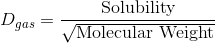Using the values from Figure 1, what is the flow rate of oxygen in terms of the flow rate of carbon dioxide, given that oxygen and carbon dioxide have equal permeability?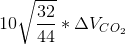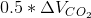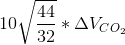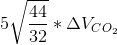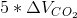Explanation:

This question is tricky because it asks you oxygen's flow rate in terms of that of carbon dioxide's; however, this problem can also be quickly solved by calculating each flow rate separately. It is important to note that since we have been told that they have equal permeability, the only values they will differ by are their partial pressure differences and molecular weights. The partial pressure difference for oxygen is 100 – 50, or 50 mmHg, and that of carbon dioxide is 50 – 40, or 10 mmHg.

From here, we see that in terms of partial pressure, the flow rate of oxygen will be 5 times greater (50/10 = 5) than carbon dioxide; however, we still must account for molecular weights, which is on the bottom of the equation. The molecular weight for oxygen is 32, and 44 for carbon dioxide; therefore, we can set our equation up to find some factor, X, that when multiplied by Pcarbon dioxide, will give us Poxygen

Poxygen = (Area/Thickness) · solubility/321/2 · 50 = (X) Pcarbon dioxide = (Area/Thickness) · solubility/441/2 · 10

Crossing out what is equal on both sides, we are left with:

50/321/2 = (X) · 10/441/2

and, X = 5 · 441/2/321/2

### Example Question #9 : Poiseuille Flow And Turbulence

Diffusion can be defined as the net transfer of molecules down a gradient of differing concentrations. This is a passive and spontaneous process and relies on the random movement of molecules and Brownian motion. Diffusion is an important biological process, especially in the respiratory system where oxygen diffuses from alveoli, the basic unit of lung mechanics, to red blood cells in the capillaries.Figure 1 depicts this process, showing an alveoli separated from neighboring cells by a capillary with red blood cells. The partial pressures of oxygen and carbon dioxide are given. One such equation used in determining gas exchange is Fick's law, given by:

ΔV = (Area/Thickness) · Dgas · (P1 – P2)

Where ΔV is flow rate and area and thickness refer to the permeable membrane through which the gas passes, in this case, the wall of the avlveoli. P1 and P2 refer to the partial pressures upstream and downstream, respectively. Further, Dgas­, the diffusion constant of the gas, is defined as:

Dgas = Solubility / (Molecular Weight)^(1/2)

Under certain conditions, alveoli enlarge or constrict. How would either change affect ΔV?

ΔV increases if enlarged, decreases if constricted.

ΔV decreases if enlarged, decreases if constricted.

Has no effect

ΔV decreases if enlarged, increases if constricted.